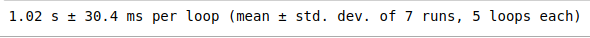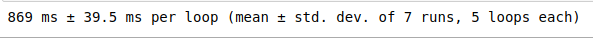# Reducing Execution time in Python using List Comprehensions

• Last Updated : 10 Jul, 2020

Prerequisites: Comprehensions in Python

Most of the competitive programmers who code in Python often face difficulty in executing the programs within the given time limit. List Comprehensions help us in reducing the execution time of a program where you are required to create a list based on any mathematical expression. We will consider an example to prove the above statement.

Attention geek! Strengthen your foundations with the Python Programming Foundation Course and learn the basics.

To begin with, your interview preparations Enhance your Data Structures concepts with the Python DS Course. And to begin with your Machine Learning Journey, join the Machine Learning - Basic Level Course

Example : To form a list of all even numbers upto 10**7, we can do it in the following ways

Method 1:

 `%``%``timeit ``-``n ``100`` ` `even ``=``[ ]``for` `i ``in` `range``(``10``*``*``7``):``    ``if` `i ``%` `2` `=``=` `0``:``        ``even.append(i)`

Output:Method 2:

 `%``%``timeit ``-``n ``100`` ` `even ``=``[i ``for` `i ``in` `range``(``10``*``*``7``) ``if` `i ``%` `2` `=``=` `0``]`

Output:We can see the difference in the run-times of the above 2 programs, this difference increases as we increase the range, also when the math expression is a bit complex. This time difference matters a lot when these type of lists are part of a very large program.

Note:The `%%timeit` tool is used which is available in Jupyter Notebook, it repeats the execution of the same cell multiple times specified by us, and returns the average/mean time taken for the execution of the given program.

Below are the Python 3 code snippets to check the execution times for the above programs,

 `import` `time `` ` ` ` `# Using for loop``start ``=` `time.time()``a ``=``[ ]`` ` `for` `i ``in` `range``(``10``*``*``7``):``    ``if` `i ``%` `2` `=``=` `0``:``        ``a.append(i)``         ` `print``(``"Execution time = "``, time.time()``-``start)`` ` `# Using list comprehension``start ``=` `time.time()``  ` `a ``=``[i ``for` `i ``in` `range``(``10``*``*``7``) ``if` `i ``%` `2` `=``=` `0``]``print``(``"Execution time = "``, time.time()``-``start)`

Output:

```Execution time =  1.558159589767456
Execution time =  0.9690220355987549```

My Personal Notes arrow_drop_up